# ZigZag (之字折线) 的力量(第二部分)。 接收、处理和显示数据的示例

28 三月 2019, 15:49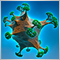0
2 798

### 概述

• 定义价格行为的指标;
• 带有图形界面的 EA，用于收集价格行为统计数据;
• 用于计算指定范围内 ZigZag 指标线段数量的 EA。

### 定义价格行为的指标

• FrequencyChangeZZ 计算相反方向 ZigZag 指标线段的形成频率。
• SumSegmentsZZ 从获得的集合中计算线段的总和及其平均值。
• PercentageSegmentsZZ 定义线段总和的百分比率和它们之间的差值。
• MultiPercentageSegmentsZZ 根据先前 PercentageSegmentsZZ 指标的数值，定义来自更高时间帧中若干线段的形成性质。

### FrequencyChangeZZ 指标

```//+------------------------------------------------------------------+
//| 填充指标缓冲区                                                      |
//+------------------------------------------------------------------+
void FillIndicatorBuffers(const int i,const datetime &time[])
{
int copy_total=1000;
for(int t=0; t<10; t++)
{
if(::CopyBuffer(zz_handle,2,time[i],copy_total,h_zz_buffer_temp)==copy_total &&
::CopyBuffer(zz_handle,3,time[i],copy_total,l_zz_buffer_temp)==copy_total &&
::CopyTime(_Symbol,_Period,time[i],copy_total,t_zz_buffer_temp)==copy_total)
{
//--- 获取 ZZ 数据
zz.GetZigZagData(h_zz_buffer_temp,l_zz_buffer_temp,t_zz_buffer_temp);
//--- 将线段集合中的柱线数量保存到指标缓冲区
segments_bars_total_buffer[i]=zz.SegmentsTotalBars();
break;
}
}
}
```

(1) 应根据所有可用数据计算数值,
(2) 形成 ZigZag 指标新线段的最小偏差
(3) 获取最终数据的极值数量。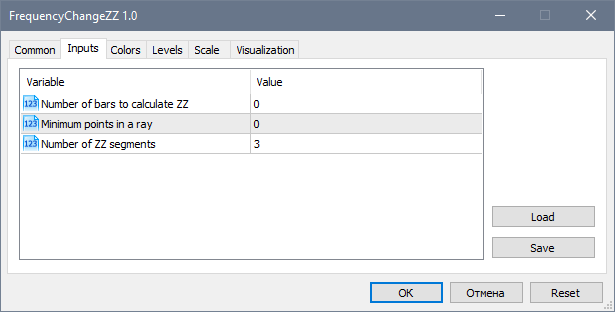FrequencyChangeZZ 指标在子窗口中显示图表，如下所示。 ZigZag 指标会被加载到主图表上以便更直观。 指标清晰显示价格在选择方向时会放缓。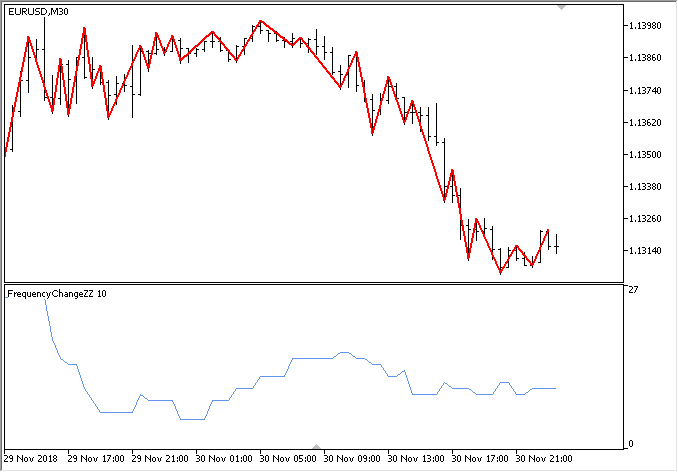### SumSegmentsZZ 指标

SumSegmentsZZ 指标中，获取数据的主要函数如下所示。 全部都与前一个示例中的相同。 唯一的区别是这里分别填充了三个指标缓冲区，分别用于向上和向下线段。 多出的一个缓冲区 用来依据当前值计算这些参数的平均值

```//+------------------------------------------------------------------+
//| 填充指标缓冲区                                                      |
//+------------------------------------------------------------------+
void FillIndicatorBuffers(const int i,const datetime &time[])
{
int copy_total=1000;
for(int t=0; t<10; t++)
{
if(CopyBuffer(zz_handle,2,time[i],copy_total,h_zz_buffer_temp)==copy_total &&
CopyBuffer(zz_handle,3,time[i],copy_total,l_zz_buffer_temp)==copy_total &&
CopyTime(_Symbol,_Period,time[i],copy_total,t_zz_buffer_temp)==copy_total)
{
//--- 获取 ZZ 数据
zz.GetZigZagData(h_zz_buffer_temp,l_zz_buffer_temp,t_zz_buffer_temp);
//--- 获取线段数据
segments_up_total_buffer[i] =zz.SumSegmentsUp();
segments_dw_total_buffer[i] =zz.SumSegmentsDown();
segments_average_buffer[i]  =(segments_up_total_buffer[i]+segments_dw_total_buffer[i])/2;
break;
}
}
}
```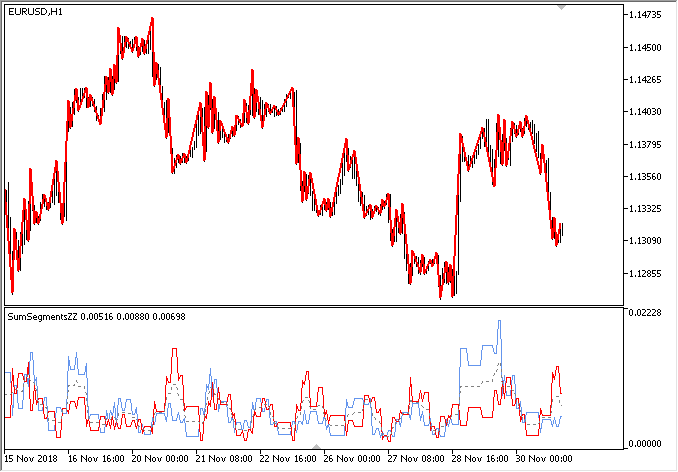### PercentageSegmentsZZ 指标

```//+------------------------------------------------------------------+
//| 填充指标缓冲区                                                      |
//+------------------------------------------------------------------+
void FillIndicatorBuffers(const int i,const datetime &time[])
{
int copy_total=1000;
for(int t=0; t<10; t++)
{
if(CopyBuffer(zz_handle,2,time[i],copy_total,h_zz_buffer_temp)==copy_total &&
CopyBuffer(zz_handle,3,time[i],copy_total,l_zz_buffer_temp)==copy_total &&
CopyTime(_Symbol,_Period,time[i],copy_total,t_zz_buffer_temp)==copy_total)
{
//--- 获取 ZZ 数据
zz.GetZigZagData(h_zz_buffer_temp,l_zz_buffer_temp,t_zz_buffer_temp);
//--- 获取线段上的数据
double sum_up =zz.SumSegmentsUp();
double sum_dw =zz.SumSegmentsDown();
double sum    =sum_up+sum_dw;
//--- 百分比和差值
if(sum>0)
{
segments_up_total_buffer[i]   =zz.PercentSumSegmentsUp();
segments_dw_total_buffer[i]   =zz.PercentSumSegmentsDown();
segments_difference_buffer[i] =fabs(segments_up_total_buffer[i]-segments_dw_total_buffer[i]);
break;
}
}
}
}
```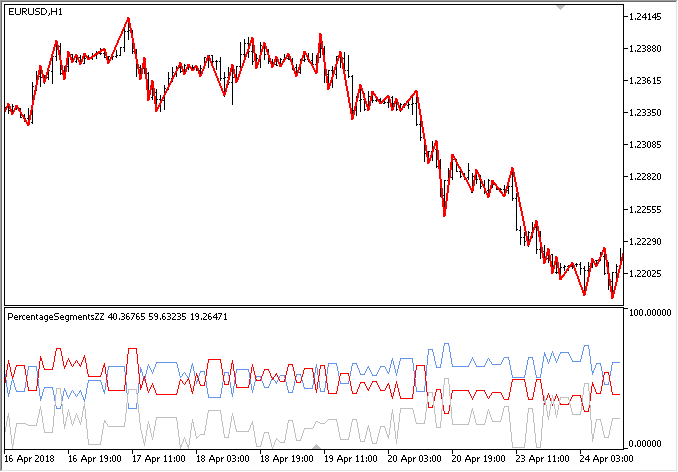### MultiPercentageSegmentsZZ 指标

```//--- 缓冲区数量
#property indicator_buffers 4
#property indicator_plots   4
//--- 缓冲区颜色
#property indicator_color1 clrSilver
#property indicator_color2 clrRed
#property indicator_color3 clrLimeGreen
#property indicator_color4 clrMediumPurple
```

```#include <Addons\Indicators\ZigZag\ZigZagModule.mqh>
CZigZagModule zz_higher_tf;
CZigZagModule zz_current0;
CZigZagModule zz_current1;
CZigZagModule zz_current2;
```

```input             int NumberOfBars    =0;         // 计算 ZZ 所需的柱线数量
input             int MinImpulseSize  =0;         // 线段的最小点数
input             int CopyExtremum    =5;         // 复制极值
input ENUM_TIMEFRAMES HigherTimeframe =PERIOD_H1; // 较高时间帧
```

```//+------------------------------------------------------------------+
//| 填充指标缓冲区                                                      |
//+------------------------------------------------------------------+
void FillIndicatorBuffers(const int i,const int total,const datetime &time[])
{
int index=total-i-1;
int copy_total=1000;
int h_buff=2,l_buff=3;
datetime start_time_in =NULL;
datetime stop_time_in  =NULL;
//--- 从更高的时间帧获取源数据
datetime stop_time=time[i]-(PeriodSeconds(HigherTimeframe)*copy_total);
CopyBuffer(zz_handle_htf,2,time[i],stop_time,h_zz_buffer_temp);
CopyBuffer(zz_handle_htf,3,time[i],stop_time,l_zz_buffer_temp);
CopyTime(_Symbol,HigherTimeframe,time[i],stop_time,t_zz_buffer_temp);
//--- 从更高的时间帧获取最终数据
zz_higher_tf.GetZigZagData(h_zz_buffer_temp,l_zz_buffer_temp,t_zz_buffer_temp);
double htf_value=zz_higher_tf.PercentSumSegmentsDifference();
//--- 第一根线段数据
zz_higher_tf.SegmentTimes(zz_handle_current,h_buff,l_buff,_Symbol,HigherTimeframe,_Period,0,start_time_in,stop_time_in);
zz_current0.GetZigZagData(zz_handle_current,_Symbol,_Period,start_time_in,stop_time_in);
//--- 第二根线段数据
zz_higher_tf.SegmentTimes(zz_handle_current,h_buff,l_buff,_Symbol,HigherTimeframe,_Period,1,start_time_in,stop_time_in);
zz_current1.GetZigZagData(zz_handle_current,_Symbol,_Period,start_time_in,stop_time_in);
//--- 第三根线段数据
zz_higher_tf.SegmentTimes(zz_handle_current,h_buff,l_buff,_Symbol,HigherTimeframe,_Period,2,start_time_in,stop_time_in);
zz_current2.GetZigZagData(zz_handle_current,_Symbol,_Period,start_time_in,stop_time_in);
//--- 基于最后一根柱线
if(i<total-1)
{
buffer_zz_higher_tf[i] =htf_value;
buffer_segment_0[i]    =zz_current0.PercentSumSegmentsDifference();
buffer_segment_1[i]    =zz_current1.PercentSumSegmentsDifference();
buffer_segment_2[i]    =zz_current2.PercentSumSegmentsDifference();
}
//--- 基于历史
else
{
//--- 如果更高时间帧有新的柱线
if(new_bar_time!=t_zz_buffer_temp)
{
new_bar_time=t_zz_buffer_temp;
//---
if(i>2)
{
int f=1,s=2;
buffer_zz_higher_tf[i-f] =buffer_zz_higher_tf[i-s];
buffer_segment_0[i-f]    =buffer_segment_0[i-s];
buffer_segment_1[i-f]    =buffer_segment_1[i-s];
buffer_segment_2[i-f]    =buffer_segment_2[i-s];
}
}
else
{
buffer_zz_higher_tf[i] =htf_value;
buffer_segment_0[i]    =zz_current0.PercentSumSegmentsDifference();
buffer_segment_1[i]    =zz_current1.PercentSumSegmentsDifference();
buffer_segment_2[i]    =zz_current2.PercentSumSegmentsDifference();
}
}
}
```

```//--- 外部参数
input            uint CopyExtremum    =3;         // 复制极值
input             int MinImpulseSize  =0;         // 最小值 impulse size
input ENUM_TIMEFRAMES HigherTimeframe =PERIOD_H1; // 较高时间帧

...

//+------------------------------------------------------------------+
//| 智能系统初始化函数                                                   |
//+------------------------------------------------------------------+
int OnInit(void)
{

...

//--- ZZ 指标的路径
string zz_path1="Custom\\ZigZag\\ExactZZ_Plus.ex5";
string zz_path2="Custom\\ZigZag\\MultiPercentageSegmentsZZ.ex5";
//--- 获取指标句柄
zz_handle_current   =::iCustom(_Symbol,_Period,zz_path1,0,MinImpulseSize,false,false);
zz_handle_higher_tf =::iCustom(_Symbol,HigherTimeframe,zz_path1,0,MinImpulseSize,false,false);
zz_handle           =::iCustom(_Symbol,_Period,zz_path2,0,MinImpulseSize,CopyExtremum,HigherTimeframe);

...

return(INIT_SUCCEEDED);
}
```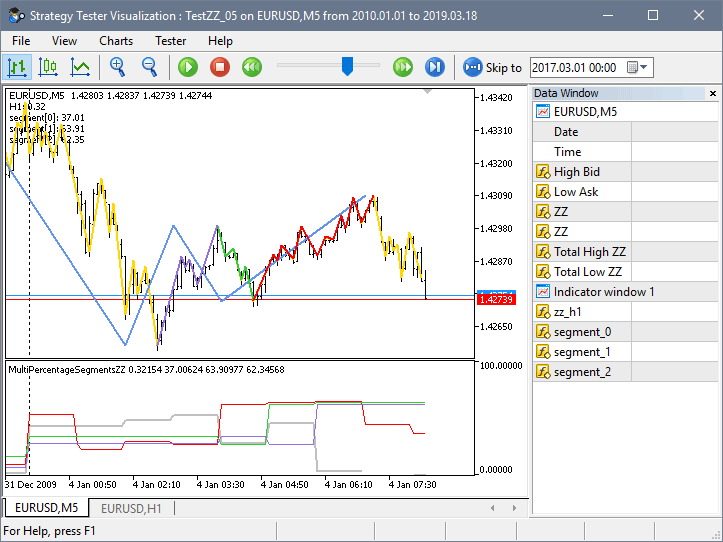### 用于收集和显示统计数据的 EA

• 更改表格每一单元格的背景颜色（CTable 类）。
• 排序方向。
• 如果启用了相应的模式，则单击表格单元格中的复选框时不会高亮显示该行。
• CKeys 类添加了数字小键盘的支持。
• 增添了 CFrame 类，用于将元素合并为组：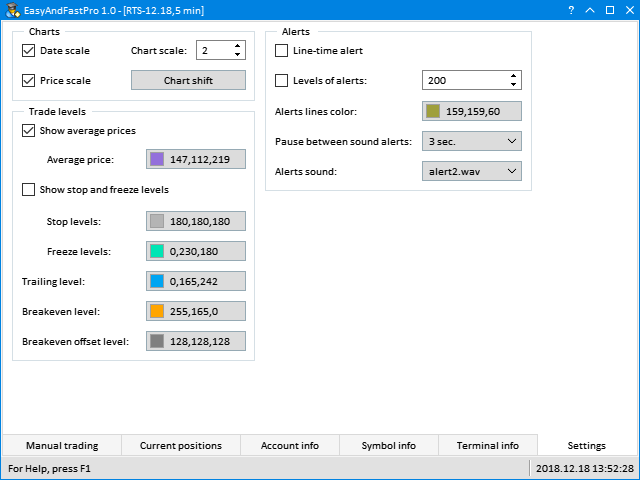• 在表格和列表中垂直滚动。
• 添加了 CWndCreate 类，其中包括用于快速创建大多数元素的基本模板方法。 它应该用作自定义类的基础。 利用这个类可令您在不同项目中无需重复声明和实现相同的创建元素方法，这极大地加速了开发进程。
• CElement 类添加了创建时元素的正确序列检查。
• CWndEvents 类中，删除元素后，ID 始终会重置。
• GetActiveWindowIndex() 方法添加到 CWndEvents，以便接收激活窗口的索引。
• 修复 CListView 类。 在 Clear() 方法中应重置某些辅助字段，以避免在 CListView 类的其他方法中超出数组范围。

• 窗体控件。
• 状态栏。
• 输入字段，用于排序市场观察窗口的列表中收集到的货币。
• 下拉日历，示意收集统计信息的开始和结束日期。
• 输入字段，用于设置指标级别。
• 数据请求按钮。
• 显示所收集数据的表格。
• 进度栏。

```//+------------------------------------------------------------------+
//|                                                      Program.mqh |
//|                                  版权所有 2018, MetaQuotes 软件公司 |
//|                                              http://www.mql5.com |
//+------------------------------------------------------------------+
#include <EasyAndFastGUI\WndCreate.mqh>
//+------------------------------------------------------------------+
//| 用于开发应用程序的类                                                 |
//+------------------------------------------------------------------+
class CProgram : public CWndCreate
{
private:
//--- 窗体
CWindow           m_window;
//--- 状态栏
CStatusBar        m_status_bar;
//--- 下拉式日历
CDropCalendar     m_from_date;
CDropCalendar     m_to_date;
//--- 按钮
CButton           m_request;
//--- 输入字段
CTextEdit         m_filter;
CTextEdit         m_level;
//--- 组合框
CComboBox         m_data_type;
//--- 表格
CTable            m_table;
//--- 进度栏
CProgressBar      m_progress_bar;
//---
public:
//--- 创建 GUI
bool              CreateGUI(void);
//---
private:
//--- 表格
bool              CreateTable(const int x_gap,const int y_gap);
};
```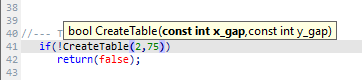```//+------------------------------------------------------------------+
//| 创建 GUI                                                          |
//+------------------------------------------------------------------+
bool CProgram::CreateGUI(void)
{
//--- 创建控制窗体
if(!CWndCreate::CreateWindow(m_window,"ZZ Market Scanner",1,1,640,480,true,true,true,true))
return(false);
//--- 状态栏
string text_items;
text_items="For Help, press F1";
int width_items[]={0};
if(!CWndCreate::CreateStatusBar(m_status_bar,m_window,1,23,22,text_items,width_items))
return(false);
//--- 货币过滤器输入字段
if(!CWndCreate::CreateTextEdit(m_filter,"Symbols filter:",m_window,0,true,7,25,627,535,"USD","Example: EURUSD,GBP,NOK"))
return(false);
else
m_filter.IsPressed(true);
//--- 下拉日历
if(!CWndCreate::CreateDropCalendar(m_from_date,"From:",m_window,0,7,50,130,D'2018.01.01'))
return(false);
if(!CWndCreate::CreateDropCalendar(m_to_date,"To:",m_window,0,150,50,117,::TimeCurrent()))
return(false);
//--- 用于指定级别的输入字段
if(!CWndCreate::CreateTextEdit(m_level,"Level:",m_window,0,false,280,50,85,50,100,0,1,0,30))
return(false);
//--- 按钮
if(!CWndCreate::CreateButton(m_request,"Request",m_window,0,375,50,70))
return(false);
//--- 表格
if(!CreateTable(2,75))
return(false);
//--- 进度栏
if(!CWndCreate::CreateProgressBar(m_progress_bar,"Processing:",m_status_bar,0,2,3))
return(false);
//--- 结束 GUI 开发
CWndEvents::CompletedGUI();
return(true);
}
```

```//+------------------------------------------------------------------+
//| 创建表格                                                           |
//+------------------------------------------------------------------+
bool CProgram::CreateTable(const int x_gap,const int y_gap)
{
#define COLUMNS1_TOTAL 4
#define ROWS1_TOTAL    1
//--- 保存指向主元素的指针
m_table.MainPointer(m_window);
//--- 列宽数组
int width[COLUMNS1_TOTAL];
::ArrayInitialize(width,50);
width=80;
//--- 沿 X 轴的列中文本偏移的数组
int text_x_offset[COLUMNS1_TOTAL];
::ArrayInitialize(text_x_offset,7);
//--- 列中的文本对齐数组
ENUM_ALIGN_MODE align[COLUMNS1_TOTAL];
::ArrayInitialize(align,ALIGN_CENTER);
align=ALIGN_LEFT;
//--- 属性
m_table.TableSize(COLUMNS1_TOTAL,ROWS1_TOTAL);
m_table.TextAlign(align);
m_table.ColumnsWidth(width);
m_table.TextXOffset(text_x_offset);
m_table.IsSortMode(true);
m_table.IsZebraFormatRows(clrWhiteSmoke);
m_table.AutoXResizeMode(true);
m_table.AutoYResizeMode(true);
m_table.AutoXResizeRightOffset(2);
m_table.AutoYResizeBottomOffset(24);
//--- 创建控件
if(!m_table.CreateTable(x_gap,y_gap))
return(false);
//--- 标题
for(uint i=0; i<m_table.ColumnsTotal(); i++)
//--- 将对象添加到公共对象组数组
return(true);
}
```

```class CProgram : public CWndCreate
{
private:
//--- 按照过滤器检查品种
bool              CheckFilterText(const string symbol_name);
};
//+------------------------------------------------------------------+
//| 按照过滤器检查品种                                                  |
//+------------------------------------------------------------------+
bool CProgram::CheckFilterText(const string symbol_name)
{
bool check=false;
//--- 如果启用了品种名称过滤器
if(!m_filter.IsPressed())
return(true);
//--- 如果输入了文本
string text=m_filter.GetValue();
if(text=="")
return(true);
//--- 切分为子串
string elements[];
ushort sep=::StringGetCharacter(",",0);
::StringSplit(text,sep,elements);
//--- 匹配检查
int elements_total=::ArraySize(elements);
for(int e=0; e<elements_total; e++)
{
//--- 删除边外空白
::StringTrimLeft(elements[e]);
::StringTrimRight(elements[e]);
//--- 如果检测到匹配
if(::StringFind(symbol_name,elements[e])>-1)
{
check=true;
break;
}
}
//--- 结果
return(check);
}
```

CProgram::GetSymbols() 方法里，循环中传递服务器上存在的所有品种，将符合指定条件的品种收集到数组中在常规循环中，所有品种都将从 “市场观察” 窗口中删除。 之后，只将数组中包含的数据添加到窗口。

```class CProgram : public CWndCreate
{
private:
//--- 品种数组
string            m_symbols[];
//---
private:
//--- 获取品种
void              GetSymbols(void);
};
//+------------------------------------------------------------------+
//| 获取品种                                                           |
//+------------------------------------------------------------------+
void CProgram::GetSymbols(void)
{
//--- 进度
m_progress_bar.LabelText("Get symbols...");
m_progress_bar.Update(0,1);
//--- 清楚品种数组
::ArrayFree(m_symbols);
//--- 收集外汇品种的数组
int symbols_total=::SymbolsTotal(false);
for(int i=0; i<symbols_total; i++)
{
//--- 获取品种名称
string symbol_name=::SymbolName(i,false);
//--- 在市场观察窗口中隐藏它
::SymbolSelect(symbol_name,false);
//--- 如果这不是外汇代码，则转到下一个
continue;
//--- 如果禁止交易，则转到下一个
continue;
//--- 按照过滤器检查品种
if(!CheckFilterText(symbol_name))
continue;
//--- 将品种保存到数组
int array_size=::ArraySize(m_symbols);
::ArrayResize(m_symbols,array_size+1,1000);
m_symbols[array_size]=symbol_name;
}
//--- 如果数组为空，则将当前品种设置为默认值
int array_size=::ArraySize(m_symbols);
if(array_size<1)
{
::ArrayResize(m_symbols,array_size+1);
m_symbols[array_size]=_Symbol;
}
//--- 在市场观察窗口中显示
int selected_symbols_total=::ArraySize(m_symbols);
for(int i=0; i<selected_symbols_total; i++)
::SymbolSelect(m_symbols[i],true);
}
```

CProgram::GetSymbolsData() 方法即用于此。 它接受两个参数：品种和时间帧。 收到指标句柄后，在指定时间范围内找出有多少根柱线存在。 可以利用应用程序的 GUI 控件指定日期范围。 接下来，我们尝试获取已计算的指标数据量。 收到句柄后，指标计算可能无法立即完成。 所以，如果 BarsCalculated() 函数返回 -1，我们要再次尝试获取有效值，直到 等于或超过指定时间范围内的总柱线数量

```class CProgram : public CWndCreate
{
private:
//--- 获取品种数据
double            GetSymbolsData(const string symbol,const ENUM_TIMEFRAMES period);
};
//+------------------------------------------------------------------+
//|  获取品种数据                                                      |
//+------------------------------------------------------------------+
double CProgram::GetSymbolsData(const string symbol,const ENUM_TIMEFRAMES period)
{
double result       =0.0;
int    buffer_index =2;
//--- 获取指标句柄
string path   ="::Indicators\\Custom\\ZigZag\\PercentageSegmentsZZ.ex5";
int    handle =::iCustom(symbol,period,path,0,0,5);
if(handle!=INVALID_HANDLE)
{
//--- 复制指定范围内的数据
double   data[];
datetime start_time =m_from_date.SelectedDate();
datetime end_time   =m_to_date.SelectedDate();
//--- 指定范围内的柱线数量
int bars_total=::Bars(symbol,period,start_time,end_time);
//--- 指定范围内的柱线数量
int bars_calculated=::BarsCalculated(handle);
if(bars_calculated<bars_total)
{
while(true)
{
::Sleep(100);
bars_calculated=::BarsCalculated(handle);
if(bars_calculated>=bars_total)
break;
}
}
//--- 获取数据
int copied=::CopyBuffer(handle,buffer_index,start_time,end_time,data);
if(copied<1)
{
while(true)
{
::Sleep(100);
copied=::CopyBuffer(handle,buffer_index,start_time,end_time,data);
if(copied>=bars_total)
break;
}

}
//--- 如果没有收到数据则退出
int total=::ArraySize(data);
if(total<1)
return(result);
//--- 计算重复次数
int counter=0;
for(int k=0; k<total; k++)
{
if(data[k]>(double)m_level.GetValue())
counter++;
}
//--- 百分比
result=((double)counter/(double)total)*100;
}
//--- 释放指标
::IndicatorRelease(handle);
//--- 返回数值
return(result);
}
```

```class CProgram : public CWndCreate
{
private:
//--- 重新构建表格
void              RebuildingTables(void);
};
//+------------------------------------------------------------------+
//| 重新构建表格                                                       |
//+------------------------------------------------------------------+
void CProgram::RebuildingTables(void)
{
//--- 删除所有行
m_table.DeleteAllRows();
//--- 添加数据
int symbols_total=::ArraySize(m_symbols);
for(int i=1; i<symbols_total; i++)
}
```

CProgram::SetData() 方法用来将数据填充至表格列内。 它需要传递两个参数（列索引和时间帧）。 在此，我们遍历指定列的单元格，在循环中用计算出的数值填充它们进度栏 显示品种和时间帧，刚刚收到的数据，以便用户了解当前正在做什么。

```class CProgram : public CWndCreate
{
private:
//--- 在指定的列设置数值
void              SetData(const int column_index,const ENUM_TIMEFRAMES period);
//--- 时间帧转化为字符串
string            GetPeriodName(const ENUM_TIMEFRAMES period);
};
//+------------------------------------------------------------------+
//| 在指定的列设置数值                                                 |
//+------------------------------------------------------------------+
void CProgram::SetData(const int column_index,const ENUM_TIMEFRAMES period)
{
for(uint r=0; r<(uint)m_table.RowsTotal(); r++)
{
double value=GetSymbolsData(m_symbols[r],period);
m_table.SetValue(column_index,r,string(value),2,true);
m_table.Update();
//--- 进度
m_progress_bar.LabelText("Data preparation ["+m_symbols[r]+","+GetPeriodName(period)+"]...");
m_progress_bar.Update(r,m_table.RowsTotal());
}
}
//+------------------------------------------------------------------+
//| 返回周期字符串对应的值                                             |
//+------------------------------------------------------------------+
string CProgram::GetPeriodName(const ENUM_TIMEFRAMES period)
{
return(::StringSubstr(::EnumToString(period),7));
}
```

```class CProgram : public CWndCreate
{
private:
//--- 在表格中填充数据
void              SetDataToTable(void);
};
//+------------------------------------------------------------------+
//| 在表格中填充数据                                                    |
//+------------------------------------------------------------------+
void CProgram::SetDataToTable(void)
{
//--- 进度
m_progress_bar.LabelText("Data preparation...");
m_progress_bar.Update(0,1);
//--- 重新构建表格
RebuildingTable();
//--- 标题
for(uint i=0; i<m_table.ColumnsTotal(); i++)
for(uint i=1; i<m_table.ColumnsTotal(); i++)
m_table.DataType(i,TYPE_DOUBLE);
//--- 在第一列里设置数值
for(uint r=0; r<(uint)m_table.RowsTotal(); r++)
m_table.SetValue(0,r,m_symbols[r],0,true);
//--- 显示表格
m_table.Update(true);
//--- 用数据填充剩余的列
SetData(1,PERIOD_M5);
SetData(2,PERIOD_H1);
SetData(3,PERIOD_H8);
}
```

```class CProgram : public CWndCreate
{
private:
//--- 获取数据
void              GetData(void);

//--- 进度（1）开始，（2）结束
void              StartProgress(void);
void              EndProgress(void);
};
//+------------------------------------------------------------------+
//| 获取数据                                                          |
//+------------------------------------------------------------------+
void CProgram::GetData(void)
{
//--- 进度开始
StartProgress();
//--- 获取品种列表
GetSymbols();
//--- 在表格中填充数据
SetDataToTable();
//--- 进度结束
EndProgress();
}
//+------------------------------------------------------------------+
//| 进度开始                                                          |
//+------------------------------------------------------------------+
void CProgram::StartProgress(void)
{
m_progress_bar.Update(0,1);
m_progress_bar.Show();
m_chart.Redraw();
}
//+------------------------------------------------------------------+
//| 进度结束                                                          |
//+------------------------------------------------------------------+
void CProgram::EndProgress(void)
{
//--- 隐藏进度栏
m_progress_bar.Hide();
//--- 更新按钮
m_request.MouseFocus(false);
m_request.Update(true);
m_chart.Redraw();
}
```

```//+------------------------------------------------------------------+
//| 事件处理器                                                         |
//+------------------------------------------------------------------+
void CProgram::OnEvent(const int id,const long &lparam,const double &dparam,const string &sparam)
{
//--- 按下按钮事件
if(id==CHARTEVENT_CUSTOM+ON_CLICK_BUTTON)
{
if(lparam==m_request.Id())
{
//--- 获取数据
GetData();
return;
}
//---
return;
}
//--- 表格排序事件
if(id==CHARTEVENT_CUSTOM+ON_SORT_DATA)
{
if(lparam==m_table.Id())
{
m_table.Update(true);
return;
}
//---
return;
}
}
```

• 启用 品种过滤器 输入字段。 它示意必须仅针对名称中包含 USD 的品种获取数据。
• 获取数据范围则在 2018.01.01 - 2018.12.21 时间段内。
• Level 的值用作计算参考点，设为 30
• 在此版本中，执行计算的时间帧在代码中严格设置：M5，H1 和 H8。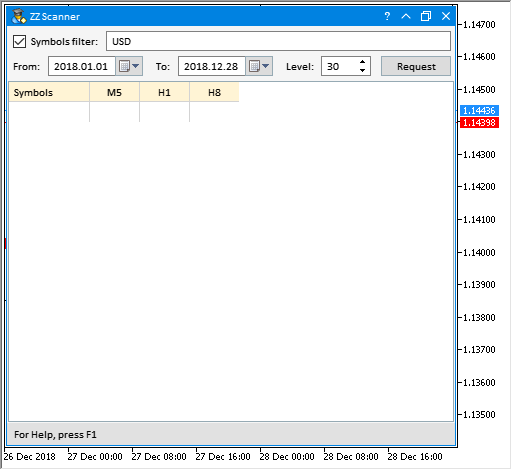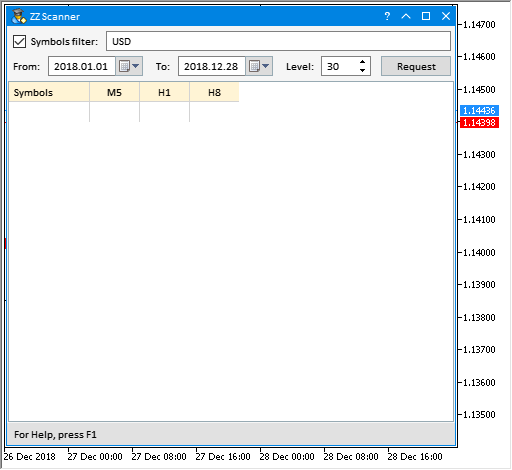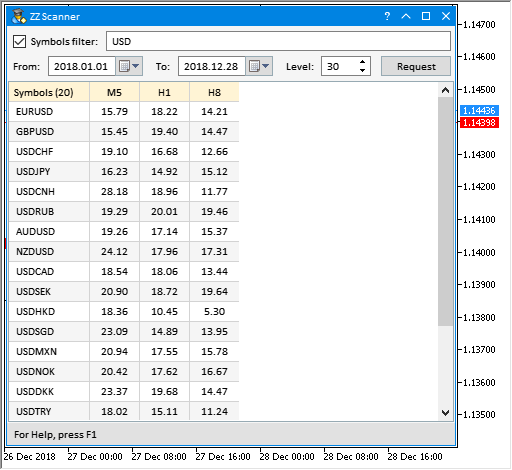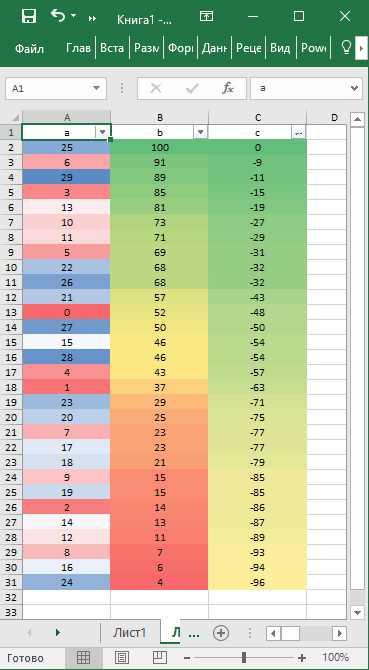`// m_table.IsZebraFormatRows(clrWhiteSmoke);`

```class CProgram : public CWndCreate
{
private:
//--- 用单元格的背景颜色填充表格
void              SetColorsToTable(void);
};
//+------------------------------------------------------------------+
//| 格式化表格                                                         |
//+------------------------------------------------------------------+
void CProgram::SetColorsToTable(void)
{
//--- 用于处理颜色
CColors clr;
//--- 用于接收渐变的数组
color out_colors[];
//--- 三色渐变
color colors={clrTomato,clrWhite,clrCornflowerBlue};
//--- 查找最低和最高表格数值
double max =0;
double min =100;
for(uint c=1; c<(uint)m_table.ColumnsTotal(); c++)
{
for(uint r=0; r<(uint)m_table.RowsTotal(); r++)
{
max =::fmax(max,(double)m_table.GetValue(c,r));
min =::fmin(min,(double)m_table.GetValue(c,r));
}
}
//--- 调整到最接近的较低整数
max =::floor(max);
min =::floor(min);
//--- 获得范围
int range =int(max-min)+1;
//--- 获得颜色渐变数组
//--- 设置单元格的背景颜色
for(uint c=1; c<(uint)m_table.ColumnsTotal(); c++)
{
for(uint r=0; r<(uint)m_table.RowsTotal(); r++)
{
int index=(int)m_table.GetValue(c,r)-(int)min;
m_table.BackColor(c,r,out_colors[index],true);
}
}
//--- 更新表格
m_table.Update();
}
```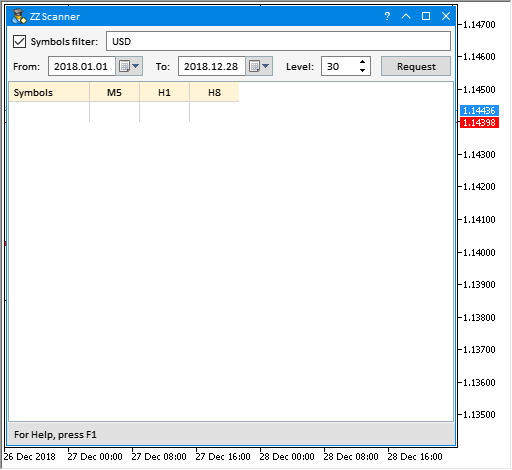### 按大小计算线段数量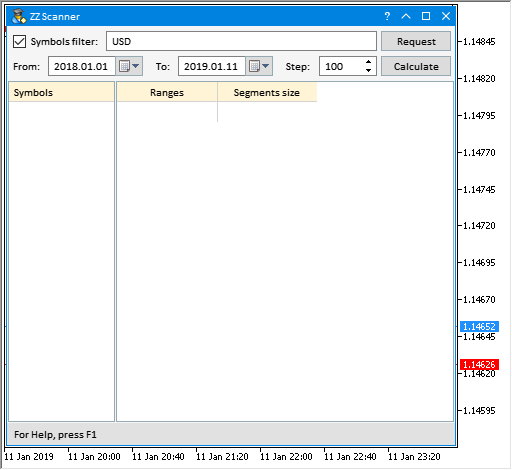```class CProgram : public CWndCreate
{
private:
//--- 获取指标数据
void              GetIndicatorData(const string symbol,const ENUM_TIMEFRAMES period);
};
//+------------------------------------------------------------------+
//| 获取指标数据                                                       |
//+------------------------------------------------------------------+
void CProgram::GetIndicatorData(const string symbol,const ENUM_TIMEFRAMES period)
{
//--- 获取指标句柄
string path   ="::Indicators\\Custom\\ZigZag\\ExactZZ_Plus.ex5";
int    handle =::iCustom(symbol,period,path,0,0);
if(handle!=INVALID_HANDLE)
{
//--- 复制指定范围内的数据
datetime start_time =m_from_date.SelectedDate();
datetime end_time   =m_to_date.SelectedDate();
m_zz.GetZigZagData(handle,2,3,symbol,period,start_time,end_time);
//--- 在状态栏中显示数据
string text="["+symbol+","+(string)GetPeriodName(period)+"] - Segments total: "+(string)m_zz.SegmentsTotal();
m_status_bar.SetValue(0,text);
m_status_bar.GetItemPointer(0).Update(true);
}
//--- 释放指标
::IndicatorRelease(handle);
}
```

```class CProgram : public CWndCreate
{
private:
//--- 数组范围
int               m_levels_array[];
//---
private:
//--- 获取级别
void              GetLevels(void);
};
//+------------------------------------------------------------------+
//| 获取级别                                                           |
//+------------------------------------------------------------------+
void CProgram::GetLevels(void)
{
//--- 释放数组
::ArrayFree(m_levels_array);
//--- 获取最大线段大小
int max_value=int(m_zz.LargestSegment()/m_symbol.Point());
//--- 用级别填充数组
int counter_levels=0;
while(true)
{
int size=::ArraySize(m_levels_array);
::ArrayResize(m_levels_array,size+1);
m_levels_array[size]=counter_levels;
//---
if(counter_levels>max_value)
break;
//---
counter_levels+=(int)m_step.GetValue();
}
}
```

CProgram::SetDataToTable2() 方法会用数据填充第二张表格。 在最开始处，将检查品种在第一张表格得列表中是否高亮显示。 如果不是，则程序退出，并发送消息到智能系统日志。 如果出于第一张表格中高亮显示行，则定义品种并获取其数据。 此后，调用上述方法接收指标数据并计算级别。 我们接收的指标数据与启动 EA 的时间帧相同。

```class CProgram : public CWndCreate
{
private:
//--- 用数据填写第二张表格
void              SetDataToTable2(void);
};
//+------------------------------------------------------------------+
//| 用数据填写第二张表格                                                 |
//+------------------------------------------------------------------+
void CProgram::SetDataToTable2(void)
{
//--- 如果不是高亮显示得行，则退出
if(m_table1.SelectedItem()==WRONG_VALUE)
{
::Print(__FUNCTION__," > Select a symbol in the table on the left!");
return;
}
//--- 进度开始
StartProgress();
//--- 隐藏表格
m_table2.Hide();
//--- 从第一张表格中获取品种
string symbol=m_table1.GetValue(0,m_table1.SelectedItem());
m_symbol.Name(symbol);
//--- 获取指标数据
GetIndicatorData(symbol,_Period);
//--- 获取级别
GetLevels();
//--- 重新构建表格
RebuildingTable2();
//--- 在第一列中设置范围
for(uint r=0; r<(uint)m_table2.RowsTotal(); r++)
m_table2.SetValue(0,r,(string)m_levels_array[r],0);
//--- 获取第二列的值
int items_total=::ArraySize(m_levels_array);
int segments_total=m_zz.SegmentsTotal();
for(int i=0; i<items_total-1; i++)
{
//--- 进度
m_progress_bar.LabelText("Get data ["+(string)m_levels_array[i]+"]...");
m_progress_bar.Update(i,m_table2.RowsTotal());
//---
for(int s=0; s<segments_total; s++)
{
int size=int(m_zz.SegmentSize(s)/m_symbol.Point());
if(size>m_levels_array[i] && size<m_levels_array[i+1])
{
int value=(int)m_table2.GetValue(1,i)+1;
m_table2.SetValue(1,i,(string)value,0);
}
}
}
//--- 显示表格
m_table2.Update(true);
//--- 结束进度
EndProgress();
}
```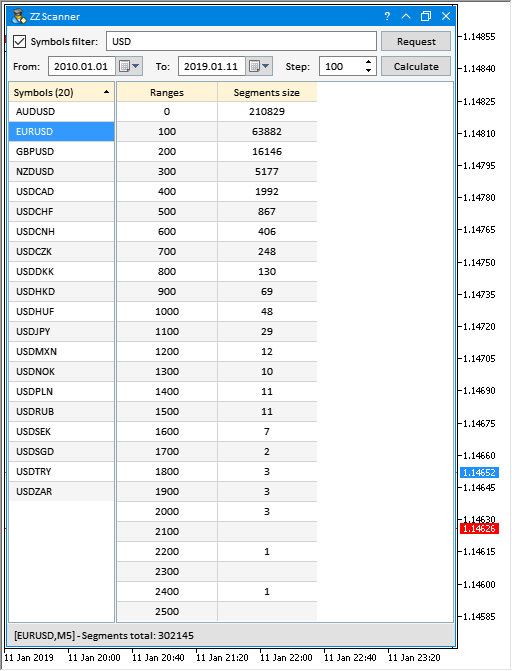### 按持续时间计算线段数量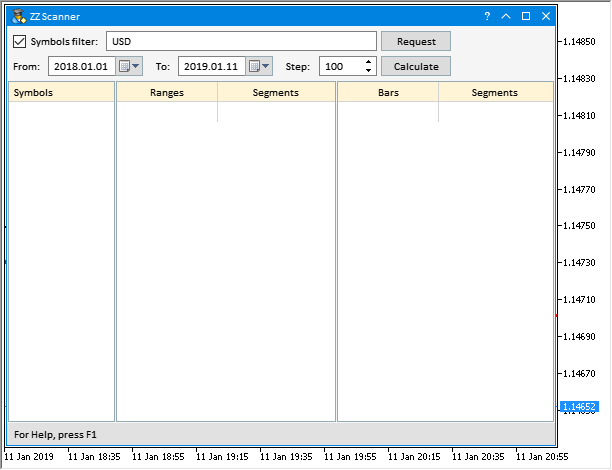• 单击 请求 以便接收品粽列表。
• 选择第一张表格中高亮行显示的品种。
• 单击 计算 以便接收第二张表格的数据。
• 若要接收第三个的数据，必要选择第二张表格中高亮显示行的范围。

```class CProgram : public CWndCreate
{
private:
//--- 用数据填充表格三
void              SetDataToTable3(void);
};
//+------------------------------------------------------------------+
//| 用数据填充表格三                                                    |
//+------------------------------------------------------------------+
void CProgram::SetDataToTable3(void)
{
//--- 如果不是高亮显示得行，则退出
if(m_table2.SelectedItem()==WRONG_VALUE)
{
::Print(__FUNCTION__," > Select a range in the table on the left!");
return;
}
//--- 进度开始
StartProgress();
//--- 隐藏表格
m_table3.Hide();
//--- 获取高亮显示的行
int selected_row_index=m_table2.SelectedItem();
//--- 范围
int selected_range=(int)m_table2.GetValue(0,selected_row_index);
//--- 重新构建表格
RebuildingTable3();
//--- 在第一列中设置数值
for(uint r=0; r<(uint)m_table3.RowsTotal(); r++)
m_table3.SetValue(0,r,(string)(r+1),0);
//--- 获取第二列的值
int segments_total=m_zz.SegmentsTotal();
for(uint r=0; r<(uint)m_table3.RowsTotal(); r++)
{
//--- 进度
m_progress_bar.LabelText("Get data ["+(string)r+"]...");
m_progress_bar.Update(r,m_table3.RowsTotal());
//---
for(int s=0; s<segments_total; s++)
{
int size =int(m_zz.SegmentSize(s)/m_symbol.Point());
int bars =m_zz.SegmentBars(s);
//---
if(size>selected_range &&
size<selected_range+(int)m_step.GetValue() &&
bars==r+1)
{
int value=(int)m_table3.GetValue(1,r)+1;
m_table3.SetValue(1,r,(string)value,0);
}
}
}
//--- 显示表格
m_table3.Update(true);
//--- 进度结束
EndProgress();
}
```

```//+------------------------------------------------------------------+
//| 事件处理器                                                         |
//+------------------------------------------------------------------+
void CProgram::OnEvent(const int id,const long &lparam,const double &dparam,const string &sparam)
{
...
//--- 单击行的事件
if(id==CHARTEVENT_CUSTOM+ON_CLICK_LIST_ITEM)
{
//--- 单击表格行
if(lparam==m_table2.Id())
{
//--- 获取第三张表格的数据
SetDataToTable3();
return;
}
//---
return;
}
...
}
```

```//+------------------------------------------------------------------+
//| 事件处理器                                                         |
//+------------------------------------------------------------------+
void CProgram::OnEvent(const int id,const long &lparam,const double &dparam,const string &sparam)
{
//--- 单击按钮的事件
if(id==CHARTEVENT_CUSTOM+ON_CLICK_BUTTON)
{
//--- 点击请求按钮
if(lparam==m_request.Id())
{
//--- 获取第一张表格的数据
SetDataToTable1();
//--- 从表格中删除不相关的数据
m_table2.DeleteAllRows(true);
m_table3.DeleteAllRows(true);
return;
}
//--- 单击计算按钮
if(lparam==m_calculate.Id())
{
//--- 获取第二张表格的数据
SetDataToTable2();
//--- 从表格中删除不相关的数据
m_table3.DeleteAllRows(true);
}
//---
return;
}
...
}
```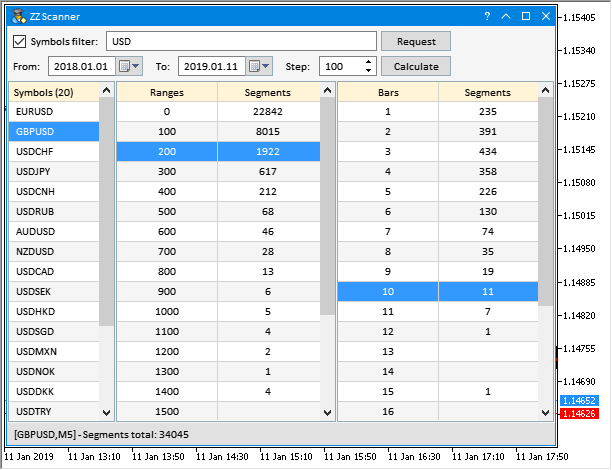### 处理图形界面的一些细节

```class CProgram : public CWndCreate
{
private:
//--- 逆初始化的最终原因
int               m_last_deinit_reason;
};
//+------------------------------------------------------------------+
//| 构造函数                                                          |
//+------------------------------------------------------------------+
CProgram::CProgram(void) : m_last_deinit_reason(WRONG_VALUE)
{
}
```

```//+------------------------------------------------------------------+
//| 逆初始化                                                          |
//+------------------------------------------------------------------+
void CProgram::OnDeinitEvent(const int reason)
{
//--- 记住最后的逆初始化原因
m_last_deinit_reason=reason;
//--- 如果原因与变更品种和周期无关，则删除 GUI
if(reason!=REASON_CHARTCHANGE)
{
CWndEvents::Destroy();
}
}
```

```//+------------------------------------------------------------------+
//| 创建 GUI                                                          |
//+------------------------------------------------------------------+
bool CProgram::CreateGUI(void)
{
//--- 如果图表或时间帧已变更，则退出
if(m_last_deinit_reason==REASON_CHARTCHANGE)
return(true);
...
return(true);
}
```

### 结束语

ZigZag 不适合生成交易信号的观念在交易论坛上广泛传播。 这有很大的误解。 事实上，没有其他指标能提供如此多的信息来判断价格行为的性质。 现在，您可以使用工具轻松获取所有必需的 ZigZag 指标数据，以便进行更详尽的分析。

MQL5\Indicators\Custom\ZigZag\FrequencyChangeZZ.mq5 用于计算反向 ZigZag 指标线段形成频率的指标
MQL5\Indicators\Custom\ZigZag\SumSegmentsZZ.mq5 用于计算所获集合的线段总合及其平均值的指标
MQL5\Indicators\Custom\ZigZag\PercentageSegmentsZZ.mq5 线段百分比总合及它们之间差值的指标
MQL5\Indicators\Custom\ZigZag\MultiPercentageSegmentsZZ.mq5 利用较高时间帧的反向线段总合的百分比之间的差值来定义若干已形成线段性质的指标
MQL5\Experts\ZigZag\TestZZ_05.mq5 用于测试 MultiPercentageSegmentsZZ 指标的 EA
MQL5\Experts\ZigZag\ZZ_Scanner_01.mq5 用于测试 PercentageSegmentsZZ 指标的 EA
MQL5\Experts\ZigZag\ZZ_Scanner_02.mq5 用于计算不同价格范围内线段的 EA
MQL5\Experts\ZigZag\ZZ_Scanner_03.mq5  用于计算位于不同价格范围，且具有不同持续时间线段的 EA

Files.zip (45.43 KB)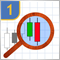研究烛条分析技术(第一部分)：检查现存形态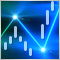ZigZag(之字折线)的力量(第一部分)。 开发指标基类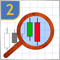研究烛条分析技术(第二部分)：自动搜索新形态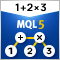利用MQL进行MQL解析